Android Games

# Android Game Development with libgdx – Jumping, Gravity and improved movement, Part 3

This is the third part in the Building a Game with LibGdx series. Make sure you read the previous articles to build a context for this one.

In the previous article we have animated Bob’s movement, but the movement is quite robotic. In this article I’ll try to make Bob jump and also move in a more natural way. I will achieve this by using a little physics. I will also clean up the code a little and fix some issues that crept into the code in the previous articles.

## Jumping – the physics

Jumping is the action performed by an entity (Bob in our case) which propels itself into the air and lands back onto the ground (substrate). This is achieved by applying a force big enough against the force exercised by the ground (gravity) on the object. Identifying the objects we have:

• Bob – entity
• Ground – substrate
• Gravity (G) – the constant force of gravity that acts on all entities in the world

To implement realistic jumping we will simply need to apply Newton’s laws of motion. If we add the necessary attributes (mass, gravity, friction) to Bob and the world we have everything we need to implement jumping. Look at the following diagram and examine its components. The left side is when we hold down the ‘jump’ button and the right side shows Bob in a jump.Let’s examine the forces in different states of Bob.

1. Bob is idle and on the ground (grounded). In this case, only the gravity acts on Bob. That means Bob is being pulled down with a constant force. The formula to calculate the force that pulls an object to the ground is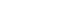where m is the mass (think weight although is not weight) and a is the acceleration. We are simplifying things and consider Bob as having a mass of 1 so the force is equal to the acceleration. If we apply a constant force to an object, its velocity increases infinitely. The formula to calculate an object’s velocity is: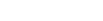where

• v – is the final velocity
• u – is the initial velocity (the velocity which t seconds ago)
• a – is the acceleration
• t – is the time elapsed since the acceleration is being applied

If we place Bob in the middle of the air that means the starting velocity is 0. If we consider that the Earth’s gravitational acceleration is 9.8 and Bob’s weight (mass) is 1 then it’s easy to calculate his falling speed after a second.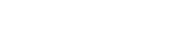So after a second in free fall, Bob accelerated from 0 to 9.8 meters per second which is 35.28 kph or 21.92 mph. That is very fast. If we want to know his velocity after a further second we would use the same formula.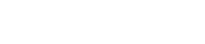That is 70.56 kph or 43.84 mph which is very fast. We already see that the acceleration is linear and that under a constant force an object will accelerate infinitely. This is in an ideal environment where there is no friction and drag. Because the air has friction and it also applies some forces to the falling object, the falling object will reach a terminal velocity at some point, past which it won’t accelerate. This depends on a lot of factors which we will ignore. Once the falling object hit the ground, it will stop, the gravity won’t affect it any more. This is not true however but we are not building a complete physics simulator but a game where Bob won’t get killed if he hits the ground at terminal velocity. Reformulating it, we check if Bob has hit the ground, and if so then we will ignore gravity.

## Making Bob jump

To make Bob jump, we need a force pointing opposite gravity (upward) which not just cancels the effect of gravity but thrusts Bob into the air. If you check the diagram, that force (F) is much stronger (its magnitude or length is much greater than that of the gravity’s vector). By adding the 2 vectors together (G and F) we obtain the final force that will act on Bob.
To simplify things, we can get rid of vectors and work only with their Y components.
On Earth,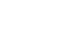. Because it is pointing down, we it’s actually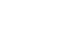. When Bob jumps, he does nothing more, than generating enough force to produce enough acceleration that will get him to height (h) before gravity (G) takes him back to the ground. Because Bob is a human like us, he can’t maintain the acceleration once he is airborne, not without a jetpack at least. To simulate this, we could create a huge force when we press the ‘jump’ key. By applying the above formulas, the initial velocity will be high enough so even if gravity will act on Bob he will still climb to a point after which he starts the free falling sequence. If we implement this method we will have a really nice realistic looking jump.

If we carefully check the original star guard game, the hero can jump to different heights depending on how long we press down the jump button. This is easily dealt with if we keep the up pointing force applied as long as we hold down the jump key and cut it off after a certain amount of time, jut to make sure that Bob does not start to fly.

## Implement Jump

I think it was enough physics, let’s see how we implement the jump. We will also do a little housekeeping task and reorganise the code. I want to isolate the jumping and movement so I will ignore the rest of the world. To see what has been modified in the code, scroll down to the Refactoring section.

Open up `BobController.java`. This is the old `WorldController.java` but was renamed. It made sense since we control Bob with it.

```public class BobController {

enum Keys {
LEFT, RIGHT, JUMP, FIRE
}

private static final long LONG_JUMP_PRESS 	= 150l;
private static final float ACCELERATION 	= 20f;
private static final float GRAVITY 			= -20f;
private static final float MAX_JUMP_SPEED	= 7f;
private static final float DAMP 			= 0.90f;
private static final float MAX_VEL 			= 4f;

// these are temporary
private static final float WIDTH = 10f;

private World 	world;
private Bob 	bob;
private long	jumpPressedTime;
private boolean jumpingPressed;

// ... code omitted ... //

public void jumpReleased() {
keys.get(keys.put(Keys.JUMP, false));
jumpingPressed = false;
}

// ... code omitted ... //
/** The main update method **/
public void update(float delta) {
processInput();

bob.getAcceleration().y = GRAVITY;
bob.getAcceleration().mul(delta);
if (bob.getAcceleration().x == 0) bob.getVelocity().x *= DAMP;
if (bob.getVelocity().x > MAX_VEL) {
bob.getVelocity().x = MAX_VEL;
}
if (bob.getVelocity().x < -MAX_VEL) {
bob.getVelocity().x = -MAX_VEL;
}

bob.update(delta);
if (bob.getPosition().y < 0) {
bob.getPosition().y = 0f;
bob.setPosition(bob.getPosition());
if (bob.getState().equals(State.JUMPING)) {
bob.setState(State.IDLE);
}
}
if (bob.getPosition().x < 0) {
bob.getPosition().x = 0;
bob.setPosition(bob.getPosition());
if (!bob.getState().equals(State.JUMPING)) {
bob.setState(State.IDLE);
}
}
if (bob.getPosition().x > WIDTH - bob.getBounds().width ) {
bob.getPosition().x = WIDTH - bob.getBounds().width;
bob.setPosition(bob.getPosition());
if (!bob.getState().equals(State.JUMPING)) {
bob.setState(State.IDLE);
}
}
}

/** Change Bob's state and parameters based on input controls **/
private boolean processInput() {
if (keys.get(Keys.JUMP)) {
if (!bob.getState().equals(State.JUMPING)) {
jumpingPressed = true;
jumpPressedTime = System.currentTimeMillis();
bob.setState(State.JUMPING);
bob.getVelocity().y = MAX_JUMP_SPEED;
} else {
if (jumpingPressed && ((System.currentTimeMillis() - jumpPressedTime) >= LONG_JUMP_PRESS)) {
jumpingPressed = false;
} else {
if (jumpingPressed) {
bob.getVelocity().y = MAX_JUMP_SPEED;
}
}
}
}
if (keys.get(Keys.LEFT)) {
// left is pressed
bob.setFacingLeft(true);
if (!bob.getState().equals(State.JUMPING)) {
bob.setState(State.WALKING);
}
bob.getAcceleration().x = -ACCELERATION;
} else if (keys.get(Keys.RIGHT)) {
// left is pressed
bob.setFacingLeft(false);
if (!bob.getState().equals(State.JUMPING)) {
bob.setState(State.WALKING);
}
bob.getAcceleration().x = ACCELERATION;
} else {
if (!bob.getState().equals(State.JUMPING)) {
bob.setState(State.IDLE);
}
bob.getAcceleration().x = 0;

}
return false;
}
}```

Take a bit of time to analyse what we have added to this class. The following lines are explained: #07 – #12 – constants containing values that affect the world and Bob

• LONG_JUMP_PRESS – time in milliseconds before the thrust applied to jump is cut off. Remember that we are doing high jumps and the longer the player presses the button the higher Bob jumps. To prevent flying we will cut off the jump propulsion after 150 ms.
• ACCELERATION – this is actually used for walking/running. It is exactly the same principle as jumping but on the horizontal X axis
• GRAVITY – this is the gravity acceleration (G pointing down in the diagram)
• MAX_JUMP_SPEED – this is the terminal velocity which we will never exceed when jumping
• DAMP – this is to smooth out movement when Bob stops. He won’t stop that sudden. More on this later, ignore it for the jump
• MAX_VEL – the same as MAX_JUMP_SPEED but for movement on the horizontal axis

#15 – this is a temporary constant and it’s the width of the world in world units. It is used to limit Bob’s movement to the screen
#19 – jumpPressedTime is the variable that cumulates the time the jump button is being pressed for
#20 – a boolean which is true if the jump button was pressed
#26 – the `jumpReleased()` has to set the `jumpingReleased` variable to false. It is just a simple state variable. Following the main update method which does most of the work for us.
#32 – calls the `processInput` as usual to check if any keys were pressed
Moving to the `processInput`
#71 – checks if the JUMP button is pressed
#72 – #76 – in case Bob is not in the `JUMPING` state (meaning he is on the ground) the jumping is initiated. Bob is set to the jumping state and he is ready for take off. We cheat a little here and instead of applying the force pointing up, we set Bob’s vertical velocity to the maximum speed he can jump with (line #76). We also store the time in milliseconds when the jump was initiated.
#77 – #85 – this gets executed whenever Bob is in the air. In case we still press the jump button we check if the time elapsed since the initiation of the jump is greater than the threshold we set and if we are still in the cut-off time (currently 150ms) we maintain Bob’s vertical speed.
Ignore lines #87-107 as they are for horizontal walking. Going back to the `update` method we have:
#34 – Bob’s acceleration is set to GRAVITY. This is because the gravity is a constant and we start from here:
#35 – we calculate the acceleration for the time spent in this cycle. Our initial values are in units/seconds so we need to adjust the values accordingly. If we have 60 updates per second then the delta will be 1/60. It’s all handled for you by libgdx.
#36 – Bob’s current velocity gets updated with his acceleration on both axis. Remember that we are working with vectors in the Euclidean space.
#37 – This will smooth out Bob’s stopping. If we have NO acceleration on the X axis then we decrease it’s velocity by 10% every cycle. Having many cycles in a second, Bobo will come to a halt very quickly but very smoothly.
#38 – #43 – making sure Bob won’t exceed his maximum allowed speed (terminal velocity). This guards agains the law that says that an object will accelerate infinitely if a constant force acts on it.
#45 – calls Bob’s `update` method which does nothing else than updates Bob’s position according to his velocity.
#46 – #66 – This is a very basic collision detection which prevents Bob to leave the screen. We simply check if Bob’s position is outside the screen (using world coordinates) and if so, then we just place Bob back to the edge. It is worth noting that whenever Bob hits the ground or reaches the edge of the world (screen), we set his status to `Idle`. This allows us to jump again.

If we run the application with the above changes, we will have to following effect:

## Housekeeping – refactoring

We notice that in the resulting application there are no tiles and Bob is not constrained only by the screen edges. There is also a different image for when Bob is in the air. One image when he is jumping and one when he is falling. We did the following:

• Renamed `WorldController` to `BobController`. It made sense since we control Bob with it.
• Commented out the `drawBlocks()` in` WorldRenderer'`s `render()` method. We don’t render the tiles now because we ignore them.
• Added the `setDebug()` method to the `WorldRendered` and the supporting toggle function in `GameScreen.java`. Debug rendering now can be toggled by pressing D on the keyboard in desktop mode.
• WorldRenderer has new texture regions to represent the jumping and falling Bob. We still maintain just one state though. How the world renderer knows when to display which, takes place by checking Bob’s vertical velocity (on the Y axis). If it’s positive, Bob is jumping, if it’s negative, Bob is falling.
```public class WorldRenderer {

// ... omitted ... //

private TextureRegion bobJumpLeft;
private TextureRegion bobFallLeft;
private TextureRegion bobJumpRight;
private TextureRegion bobFallRight;

TextureAtlas atlas = new TextureAtlas(Gdx.files.internal('images/textures/textures.pack'));

// ... omitted ... //

bobJumpLeft = atlas.findRegion('bob-up');
bobJumpRight = new TextureRegion(bobJumpLeft);
bobJumpRight.flip(true, false);
bobFallLeft = atlas.findRegion('bob-down');
bobFallRight = new TextureRegion(bobFallLeft);
bobFallRight.flip(true, false);
}

private void drawBob() {
Bob bob = world.getBob();
bobFrame = bob.isFacingLeft() ? bobIdleLeft : bobIdleRight;
if(bob.getState().equals(State.WALKING)) {
bobFrame = bob.isFacingLeft() ? walkLeftAnimation.getKeyFrame(bob.getStateTime(), true) : walkRightAnimation.getKeyFrame(bob.getStateTime(), true);
} else if (bob.getState().equals(State.JUMPING)) {
if (bob.getVelocity().y > 0) {
bobFrame = bob.isFacingLeft() ? bobJumpLeft : bobJumpRight;
} else {
bobFrame = bob.isFacingLeft() ? bobFallLeft : bobFallRight;
}
}
spriteBatch.draw(bobFrame, bob.getPosition().x * ppuX, bob.getPosition().y * ppuY, Bob.SIZE * ppuX, Bob.SIZE * ppuY);
}
}```

The above code excerpt shows the important additions.
#5-#8 – The new texture regions for jumping. We need one for left and one for right.
#15-#20 – The preparation of the assets. We need to add a few more png images to the project. Check the `star-assault-android/images/` directory and there you will see `bob-down.png` and `bob-up.png`. These were added and also the texture atlas recreated with the `ImagePacker2` tool. See Part 2 on how to create it.
#28-#33 – is the part where we determine which texture region to draw when Bob is in the air.

• There were some bug fixes in `Bob.java`. The bounding box now has the same position as bob and the update takes care of that. Also the `setPosition` method updates the bounding boxes’ position. This had an impact on the `drawDebug()` method inside the `WorldRenderer`. Now we don’t need to worry about calculating the bounding boxes based on the tiles’ position as the boxes now have the same position as the entity. This was a stupid bug which I let to slip in. This will be very important when doing collision detection.

This list pretty much sums up all the changes but it should be very easy to follow through. The source code for this project can be found here: https://github.com/obviam/star-assault You need to checkout the branch part3. To check it out with git: `git clone -b part3 git@github.com:obviam/star-assault.git` You can also download it as a zip file.

Reference: Android Game Development with libgdx – Jumping, Gravity and improved movement, Part 3 from our JCG partner Impaler at the Against the Grain blog.

## Want to know how to develop your skillset to become a Java Rockstar?#### and many more ....